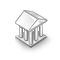Interesting script?
So post a link to it -
let others appraise it

You liked the script? Try it in the MetaTrader 5 terminal# Doubles comparer - library for MetaTrader 5

Views:
603
Rating:
Published:
2020.06.10 12:54
Updated:
2020.06.13 12:55

A tiny class to compare floating point variables the right way with desired precision.

```template<typename T>class FloatingPoint
{
public:
FloatingPoint() {Precision(sizeof(T));}
int               Compare(const T x, const T y) {return (((fabs(x-y)<min))?0:((x-y>=min))?1:-1);}
void              Precision(int digits) {min=pow(10,-fabs(digits));}
protected:
double            min;
};
```

Let's say you have two doubles with a value of 1.15.

When you compare them, you expect them to be equal.

But doubles in computer behave the strange way.

1.15 may be stored as 1.14999999999.

So comparing will fail your expectations.

The proper way to compare floating point variables, is by checking their subtraction with some minimum value.

FlatingPoint must be initialized with double or float datatype.

Comparer method returns 0 if x = y; 1 if x > y; and -1 if x < y.

Precision can be changed.

Deafult precision is 8 digits after fp for doubles, and 4 for floats.

Example of FloatingPoint class usage.

```void OnStart()
{
FloatingPoint<double>fp;
double a=1.15,b=1.14999999999;
for(int i=15; i>5; i--)
{
fp.Precision(i);
int compare=fp.Compare(a,b);
string res=(compare==0)?"=":(compare==1)?">":"<";
PrintFormat("%g %s %g at %d digit precision",a,res,b,i);
}
}
```

Output:

```/**
you do not expect this:
1.15 > 1.15 at 15 digit precision
1.15 > 1.15 at 14 digit precision
1.15 > 1.15 at 13 digit precision
1.15 > 1.15 at 12 digit precision
1.15 > 1.15 at 11 digit precision

you expect this:
1.15 = 1.15 at 10 digit precision
1.15 = 1.15 at 9 digit precision
1.15 = 1.15 at 8 digit precision
1.15 = 1.15 at 7 digit precision
1.15 = 1.15 at 6 digit precision
/**/
```WaveMTF MT5

Indicator WaveMTF Bull and Bear System with Signal and Alert for MetaTrader 5 with options to display signal on the chart.Basket Closer Profit/LossArrays classBar Time Count Down